# N-Factor Calculation - Redox Reactions Chemistry Notes | EduRev

## Chemistry : N-Factor Calculation - Redox Reactions Chemistry Notes | EduRev

The document N-Factor Calculation - Redox Reactions Chemistry Notes | EduRev is a part of the Chemistry Course Physical Chemistry.
All you need of Chemistry at this link: Chemistry

6.  n-FACTOR CALCULATION

6.1  Acids

Acids are the species which furnish Hions when dissolved in a solvent. For acids, n-factor is defined as the number of H+ ions replaced by 1 mole of acid in a reaction. Note that the n-factor for acid is not equal to its basicity; i.e. the number of moles of replaceable H+ atoms present in one mole of acid.

For example, n-factor of HCl = 1

n-factor of HNO3 = 1

n-factor of H2SO4 = 1 or 2, depending upon extent of reaction it undergoes.

H2SO+ NaOH → NaHSO4 + H2O

Although one mole of H2SO4 has 2 replaceable H atoms but in this reaction H2SO4 has given only one H+ ion, so its n-factor would be 1.

H2SO+ 2NaOH → Na2SO4 + 2H2O

The n-factor of H2SO4 in this reaction would be 2 .

Similarly ,        n-factor of H2SO3 = 1 or 2

n-factor of H2CO3 = 1 or 2

n-factor of H3PO4 = 1 or 2 or 3

n-factor of H3PO3 = 1 or 2 because one of the H is not replaceable in H3PO. this can be seen using it structure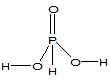. The H atoms which are linked to oxygen are replaceable whicle the H atom linked directly to central atom (P) is non replaceable.

n-factor of H3BO3 = 1

In H3BOalthough all three H are linked to oxygen, yet all three H are not replaceable. Here, boron atom is electron deficient, so it acts as a Lewis Acid . When H3BO3 is added to water , then oxygen atom of H2O through its lone pair attack the boron atom, as follows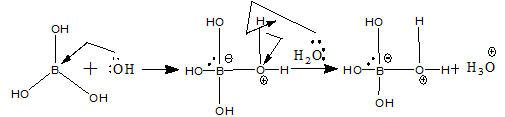The net reaction is H3BO3 + 2H2O → [B(OH)4]- + H3O+ .

Thus, one mole of H3BOin solution gives only one mole of H+ , so its n-factor is 1.

6.2 Bases

Bases are the species, which furnish OH- ions when dissolved in a solvent. For bases, n-factor is defined as the number of OH- ions replaced by 1 mole of base in a reaction. Note that n-factor is not equal to its acidity i.e. the number of moles of replaceable OH- ions present in 1 mole of base.

For example, n-factor of NaOH = 1

n-factor of Zn(OH)2 = 1 or 2

n-factor of Ca(OH)2 = 1 or 2

n-factor of Al(OH)3 = 1 or 2 or 3

n-factor of NH4(OH) = 1

6.3 SALTS WHICH REACT SUCH THAT NO ATOM UNDERGOES CHANGE IN

OXIDATION STATE

The n-factor for such salts is defined as the total moles of cationic / anionic charge replaced in one mole of the salt. For the reaction,

Na3PO4 + BaCl2 → NaCl + Ba3(PO4)2

To get one mole of Ba3(PO4)2, two moles of  Na3POare required, which means six moles of Na+ are completely replaced by 3 moles of Ba2+ ions. So, six moles cationic charge is replaced by 2 moles of Na3PO, thus each mole of Na3PO4 replaces 3 moles of cationic charge. Hence, n-factor of Na3PO4 in this reaction is 3.

6.4 SALTS WHICH REACT IN A MANNER THAT ONLY ONE ATOM UNDERGOES

CHANGE IN OXIDATION STATE AND GOES IN ONLY ONE PRODUCT

The n-factor of such salts is defined as the number of moles of electrons exchanged
( lost or gained )by one mole of the salt.

Let us have a salt AaBb in which oxidation state of A is +x. it changes to a compound, which has atom D in it. The oxidation state of A is AcD be +y.

Aa+xB→Ac+yD

The n-factor of AaBis calculated as n = |ax - ay|

To calculate n-factor of a salt of such type, we take one mole of the reactant and find the number of mole of the element whose oxidation state is changing. This is multiplied with the oxidation state of the element in the reactant which gives us the total oxidation state of the element in the reactant. Now we calculate the total oxidation state of the same element in the product for the same number of mole of atoms of that element in the reactant. Remember that the total oxidation state of the same element in the product is not calculated for the number of mole of atoms of that element in the product.

For example, let us calculate the n-factor of KMnO4 for the given chemical change.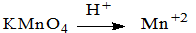In this reaction, oxidation state of Mn changes from +7 to +2 . Thus, KMnO4 is acting as oxidizing agent, since it is reduced.

n-factor of KMnO4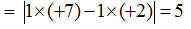Similarly,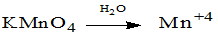n-factor of KMnO4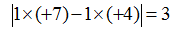and ,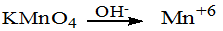n-factor of KMnO4 =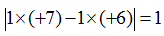It can be seen that in all the above chemical changes, KMnO4 is acting as oxidizing agent, yet its n-factor is not same in all reactions. Thus, the n-factor of a compound is not fixed, it depends on the type and the extent of reaction it undergoes.

6.5 SALTS WHICH REACT IN A MANNER THAT ONLY ONE ATOM UNDERGOES CHANGE IN OXIDATION STATE BUT GOES IN TWO PRODUCTS WITH THE  SAME OXIDATION STATE

Let us have a salt AaBb in which oxidation state of A is –x. It undergoes a reaction such that element A changes its oxidation state and goes in more than one ( two ) products with the same oxidation state ( but different oxidation state than in the reactant ). In such case, the n-factor is calculated in the same manner as in case 4

Let the chemical change be.

Aa+xB→Ac+yD + Ae+yF

In such cases, the number of products in which element A is present is of no significance since the oxidation state of A in both the products is same. The point of important is not the number of products containing that element which undergoes change in oxidation state but the oxidation state of the element is of importance. The n-factor of AaB is calculated in the same way as in case 4.

The n-factor of AaBis calculated as n = |ax - ay|

For example let us calculate the n-factor of K2Cr2O7 for the given chemical change

Cr2O72– → Cr3+ + Cr3+

In this reaction, oxidation state of Cr changes from +6 to +3 in both the products.

n-factor of K2Cr2O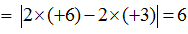6.6 SALTS WHICH REACT IN A MANNER THAT ONLY ONE ATOM UNDERGOES CHANGE IN OXIDATION STATE BUT GOES IN TWO PRODUCTS WITH THE DIFFERENT OXIDATION STATE ( DIFFERENT THAN IN THE REACTANT )

AS A RESULT OF EITHER OXIDATION OR REDUCTION

Let the chemical change be

Aa+xB→Ac+yD + Ae+zF

In such cases, n- factor calculation is not possible until we know that how much of A has changed its oxidation state to +y and how much of A has changed its oxidation state to +z. This is because the number of moles of electrons lost or gained by one mole of AaBwould depend on the fact that how much of A underwent change to oxidation state +y and how much of A underwent change to oxidation state +z. This is possible only by knowing the balanced chemical reaction. If we know the balanced chemical reaction, then the n-factor calculation is of no use because problem can be solved using mole concept. But nevertheless , n-factor calculation in such cases can be done as follows

Let us take a chemical change   2Mn+7 → Mn+4 + Mn+2   out of the two moles of Mn+7 , one mole of Mn+7 changes to Mn+4 by gaining 3 moles of electrons and other mole of Mn+7 changes to Mn+2 by gaining 5 mole of electrons, so in all 8 mole of electrons are gained by 2 mole of Mn+7 . So each mole of Mn+7 has gained 8/2 = 4 mole of electrons. Thus, 4 would be the n-factor of Mn+7 in this reaction.

If the reaction would have been ,

3Mn+7 → Mn+4 + 2Mn+2

Out of three moles of Mn+7 , two moles of Mn+7 changes to Mn+2 by gaining 10 moles of electrons and one mole of Mn+7 changes to Mn+4 by gaining 3 mole of electrons . So each mole of Mn+7 has gained 13/3 mole of electrons. Thus, 13/3 would be the n-factor of Mn+7 in this reaction.

Note that n-factor can be a fraction because it is not the number of electrons exchanged but it is the number of mole of electrons exchanged which can be a fraction.

Now if the reaction would have been  3Mn+7 → 2Mn+4 + Mn+2. Thus, each mole Mn+7 have gained 11/3 mole of electrons. Thus, 11/3 would be the n-factor of Mn+7 in this reaction.

6.7 SALTS WHICH REACT IN A MANNER THAT ONLY ONE ATOM UNDERGOES CHANGE IN OXIDATION STATE BUT GOES IN TWO PRODUCTS WITH THE DIFFERENT OXIDATION STATE ( IN ONE PRODUCT WITH THE SAME OXIDATION STATE THE REACTANT AND IN OTHER PRODUCT WITH THE DIFFERENT OXIDATION STATE THAN IN THE REACTANT)

Let the reaction be

Aa+xB→Ae+xF+ Ac+yD

For such reactions also, the n-factor calculation is not possible without the knowledge of balanced chemical reaction because n-factor of AaBwould depend on the fact that how much of A underwent change to oxidation state +y and how much of A remained in the same oxidation state +x.

For example, if we have a chemical change as

2Mn+7 → Mn+7 + Mn+2 (the compounds containing Mn in +7 state in reactant and product are different )

In this reaction, 5 moles of electrons are gained by 2 moles of Mn+7, so each mole of Mn+7 takes up 5/2 moles of electrons. Therefore, n-factor of Mn+7 in this reaction would be 5/2.

6.8  SALTS WHICH REACT IN A MANNER THAT TWO TYPE OF ATOM

UNDERGO CHANGE IN OXIDATION STATE (BOTH THE ATOMS ARE

EITHER GETTING OXIDIZED OR REDUCED)

Let the change be given as

Aa+xB→Ac+yD+ EfB+z

in this reaction, both A and B are changing their oxidation states and both of them are either getting oxidized or reduced. In such cases, n-factor of the compound would be the sum of individual n-factors of A and B .

n-factor of A = |ax - ay|

n-factor of B = |ax - bz| because the total oxidation state of ‘b’ B’s in the reactant is –ax ( as total oxidation of ‘a’ A’s in the reactant is +ax) and the total oxidation state of y B’s in the product is bz.

n-factor of AaBb =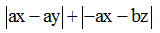In general, the n-factor of the salt will be the total number of mole of electrons lost or gained by one mole of the salt.

For example, we have a reaction,

Cu2+1S-2 →Cu2+ + S+4O2

In which Cu+ and S2- both are getting oxidized to Cu2+ and S+4 respectively.

N factor of Cu2S =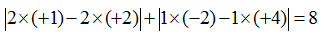6.9      SALTS WHICH REACT IN A MANNER THAT TWO ATOMS

UNDERGOES CHANGE IN OXIDATION STATE ( ONE ATOM IS

GETTING OXIDIZED AND THE OTHER IS GETTING REDUCED)

If we have a salt which react in a fashion that atoms of one of the element are getting oxidized and the atoms of another element are getting reduced and no other element on the reactant side is getting oxidized or reduced, then the n-factor of such a salt can be calculated either by taking the total number of moles of electrons lost or total number of moles of electrons gained by one mole of the salt.

For example, the decomposition reaction of KClO3 is given as

KCl+5O32- → KCl-1 + O20

In this reaction, O2- is getting oxidized to O2 and KCl+5 is getting reduced to KCl-1 . in each case, 6 moles of electrons are exchanged whether we consider oxidation or reduction.

n-factor of KClOconsidering oxidation =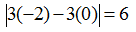n-factor of KClOconsidering reduction =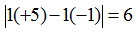6.9 SALTS OR COMPOUNDS WHICH UNDERGO DISPROPORTIONATION REACTION)

Disproportionation reactions are the reactions in which oxidizing and reducing agents are the same or the same element from the same compound is getting oxidized as well as reduced.
n-factor of a disproportionation reaction can only be calculated using a balanced chemical reaction. We will categorize disproportionation reaction into two types.

(a)  Disproportionation reactions in which moles of compound getting oxidized and reduced are same i.e. moles of oxidizing agent and reducing agent are same. Then n-factor for such compounds is calculated by either the number of moles of electrons lost or gained by one mole of the compound because in such a case, n-factor of the compound acting as oxidizing agent or as reducing agent would be same.

For example, 2H2O2 2H2O +O2

Out of the two moles of H2Oused in the reaction, one mole of H2Ogets oxidized to O(oxidation state of O changes from -1 to 0 ) while the other mole of H2O2 gets reduced to H2O ( the oxidation state of O changes from -1 to -2 ). When one mole of H2O2 gets oxidized to O2  ,the half reaction would be     O22- → O20 + 2e-

And when one mole of H2O2 gets reduced to H2O, the half-reaction would be

O22- + 2e-→  2O2-

Thus, it is evident that one mole of H2O(which is either getting oxidized or reduced ) will lose or gain 2 mole of electrons. Therefore, n-factor of H2Oas oxidizing agent as well as reducing agent in this reaction is 2. Thus,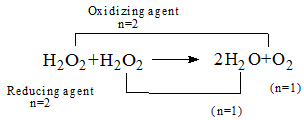Or when the reaction is written as 2H2O2 → 2H2O +O2 ,where, H2O is not distinguished as how much of it functions as oxidizing agent and how much as reducing agent, then n-factor calculation can be done in the following manner. Find the number of electrons exchanged ( lost or gained ) using the balanced equation and divide it by the number of moles of H2O2 involved in the reaction. Thus, the n-factor of H2O2 when the reaction is written without segregating oxidizing agent and reducing agent is 2/2 = 1.

2H2O2     →    2H2O     +    O2

(n=1)             (n=1)          (n=2)

(b) Disproportionation reaction in which moles of compound getting oxidized and reduced are not the same i.e. moles of oxidizing agent and reducing agent are not the same. For example

6Br2 + 12OH-   → 10Br- + 2BrO3- + 6H2O

In this reaction , the mole of electrons lost by the oxidation of some of the moles of Br2 are same as the number of mole of electrons gained by the reduction of rest of the moles Br2. Of the 6 moles of Br2 used, one mole is getting oxidized, loosing 10 electrons ( as reducing agent ) and 5 moles of Br2 are getting reduced and accepts 10 moles of electrons ( as oxidizing agent ).

Br2  →  2Br+5 + 10e-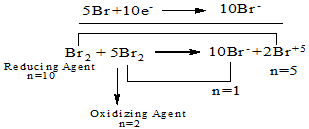Thus, n-factor of Br2 acting as oxidizing agent is 2 and that Br2 acting as reducing agent has
n-factor 10.

Or when the reaction is written as

6Br2    → 10Br- + 2Br+5

Where, Bris not distinguished as how much of it functions as oxidizing agent and how much of it as reducing agent, then for calculating n-factor of compound in such reactions, first find the total number of mole of electrons exchanged ( lost or gained ) using the balanced equation and divide it with the number of moles of Brinvolved in the reaction to get the number of moles of electrons exchanged by one mole of Br2 .

In the overall reaction, the number of moles of electrons exchanged ( lost or gained ) is 10 and the moles of Brused in the reaction are 6. Thus, each mole of Brhas exchanged 10/6 or 5/3 moles of electrons. Therefore, the n-factor of Br2 when the reaction is written without segregating oxidizing and reducing agent is 5/3.

6Br2    → 10Br- + 2Br+5

(n=5/3)       (n=1)   (n=5)

Offer running on EduRev: Apply code STAYHOME200 to get INR 200 off on our premium plan EduRev Infinity!

## Physical Chemistry

77 videos|83 docs|32 tests

,

,

,

,

,

,

,

,

,

,

,

,

,

,

,

,

,

,

,

,

,

;